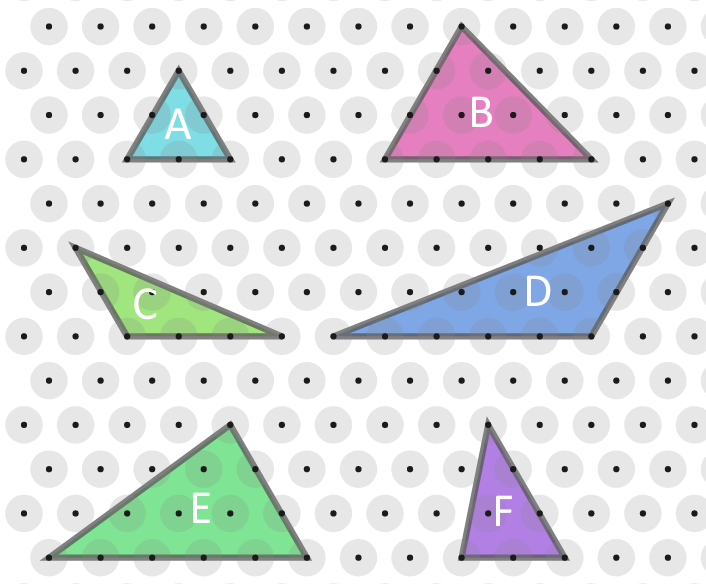#### You may also like### Kissing Triangles

Determine the total shaded area of the 'kissing triangles'.### Isosceles

Prove that a triangle with sides of length 5, 5 and 6 has the same area as a triangle with sides of length 5, 5 and 8. Find other pairs of non-congruent isosceles triangles which have equal areas.Four rods, two of length a and two of length b, are linked to form a kite. The linkage is moveable so that the angles change. What is the maximum area of the kite?

# More Isometric Areas

##### Age 11 to 14Challenge Level

This problem follows on from Isometric Areas.
You may wish to print off some isometric paper.

Here is an equilateral triangle with sides of length 1.
Let's define a unit of area, $T$, such that the triangle has area $1T$.Each of the triangles below has at least two edges whose side lengths are whole numbers.
For example triangle $B$ has sides of length $3$ and $4$.Work out the area, in terms of $T$, of each of the triangles.

Compare the areas to the whole number side lengths.
What do you notice?
Can you explain what you have noticed?

You might like to try Of All the Areas next.